# Introduction..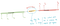Illustration of Bottleneck issue, generated by author

So it clearly seems that the final hidden state is acting some sort of bottleneck. As the input sequence length of the input increases, encoding the entire information in that context vector becomes infeasible. The attention mechanism solves this bottleneck problem in a way that decoder knows not only the final hidden state but hidden states of all encoder time steps. In this blog, we will discuss about the attention mechanism and how it solves for bottleneck issue.

# Attention Mechanism..

To achieve this, the idea of attention is to create a context vector “Ci” which is weighted sum of all the encoder hidden states. So the context vector is not static anymore, we will have different context vectors at each time step of decoding. The context vector “Ci” is generated anew at each decoding step i by applying weighted sum of all the hidden states of encoder.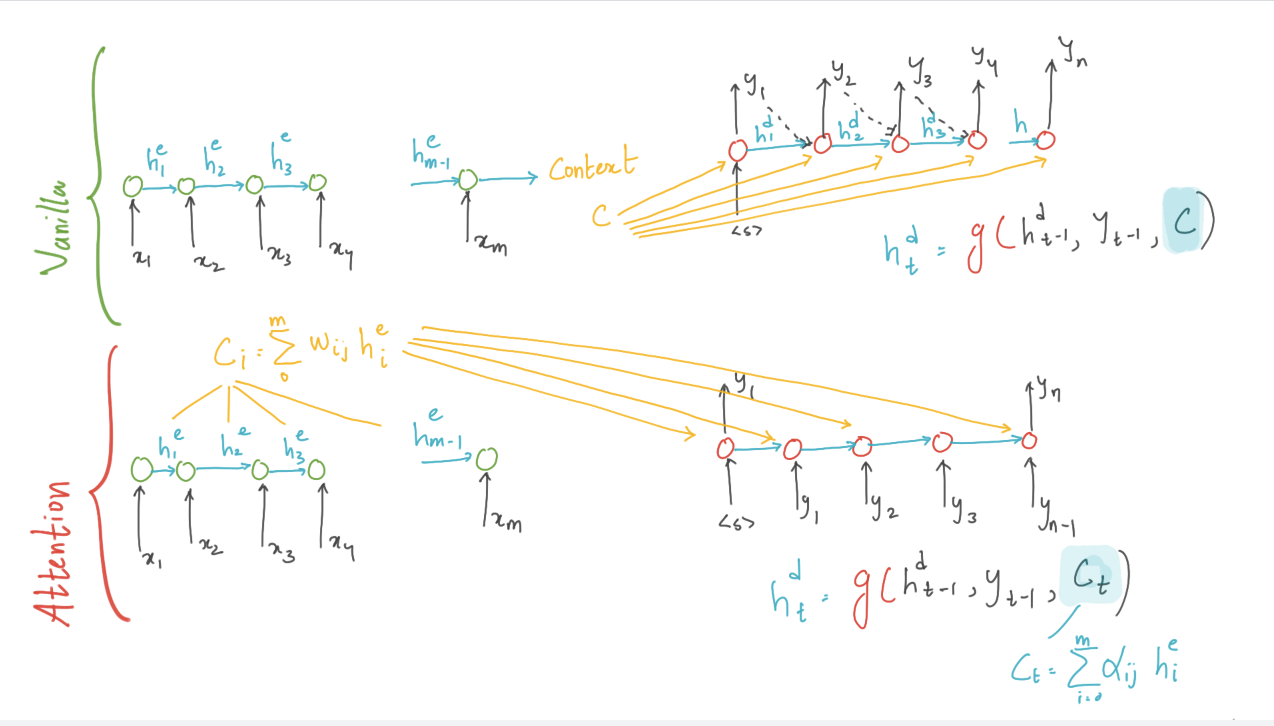illustration of vanilla and attention encoder-decoder model, generated by author

## Weights of Attention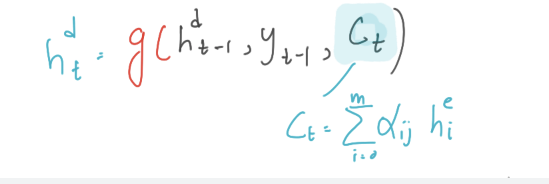hidden state calculation at decoding step t, generated by author

So at each time step of decoding, we compute a context “ct” which is made available for computing the decoding hidden state at time step t. The first step in computing ct is to compute the weights/relevance on each encoder state. The weight can be seen as a score of relevance that each encoder state hj that has while computing the decoder at time step t.

## Dot-Product Attention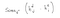Score equation, generated by author

The output from this dot product gives us the degree of similarity, this score across all the hidden states will give us the relevance of every encoder state to the current step of decoder. We will have to normalise the scores to get the weights.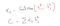Dynamic context vector equation, generated by author

Now we finally arrived at a method to calculate the dynamic context vectors that takes into account from the entire hidden states while encoding the input sequence. Dot product attention helps us understand the essence of attention mechanism itself. But its also possible to create more sophisticated scoring function. We will discuss them briefly in coming sections

# Note:

RL | ML | ALGO TRADING | TRANSPORTATION | GAME THEORY

## More from Kowshik chilamkurthy

RL | ML | ALGO TRADING | TRANSPORTATION | GAME THEORY